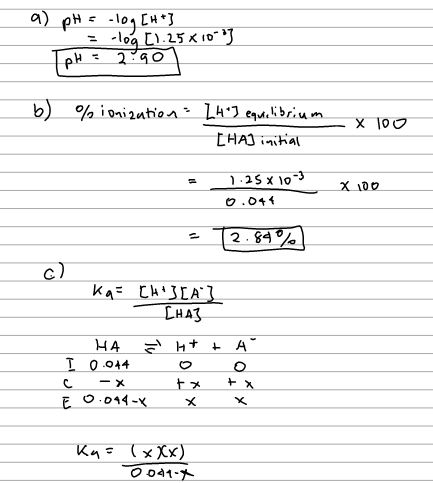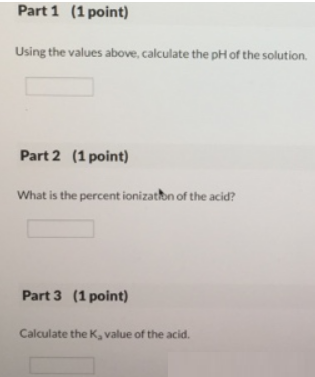# Problem: Part 1A 0.0440 M solution of in organic acid has an [H+] of 1.25 x 10-3 M. Using the values above, calculate the pH of the solution. Part 2What is the percent ionization of the acid? Part 3Calculate the Ka value of the acid.

###### FREE Expert Solution93% (101 ratings)###### Problem Details

Part 1

A 0.0440 M solution of in organic acid has an [H+] of 1.25 x 10-3 M. Using the values above, calculate the pH of the solution.

Part 2

What is the percent ionization of the acid?

Part 3

Calculate the Ka value of the acid.